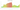# ZK

## Zambian Kwacha

Zambia uses the Kwacha as its currency. 100 Ngwee are broken up into it.

#### how has the value of the currency changed in the last year?## USD 1 = ZMW 19.104

How much is10 US dollars worth inZambian kwachas?
At the current exchange rate, 10 US dollars is worth 191.04 Zambian kwachas
How much is50 US dollars worth inZambian kwachas?
At the current exchange rate, 50 US dollars is worth 955.2 Zambian kwachas
How much is100 US dollars worth inZambian kwachas?
At the current exchange rate, 100 US dollars is worth 1,910.4 Zambian kwachas
How much is500 US dollars worth inZambian kwachas?
At the current exchange rate, 500 US dollars is worth 9,552 Zambian kwachas
How much is2,000 US dollars worth inZambian kwachas?
At the current exchange rate, 2,000 US dollars is worth 38,208.01 Zambian kwachas
How much is10 US dollars worth inZambian kwachas?
At the current exchange rate, 10 US dollars is worth 191.04 Zambian kwachas
How much is50 US dollars worth inZambian kwachas?
At the current exchange rate, 50 US dollars is worth 955.2 Zambian kwachas
How much is100 US dollars worth inZambian kwachas?
At the current exchange rate, 100 US dollars is worth 1,910.4 Zambian kwachas
How much is500 US dollars worth inZambian kwachas?
At the current exchange rate, 500 US dollars is worth 9,552 Zambian kwachas
How much is2,000 US dollars worth inZambian kwachas?
At the current exchange rate, 2,000 US dollars is worth 38,208.01 Zambian kwachas